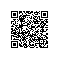# 由服务器IP判断说起的攻防——站长安全顾问系列话题一

number.inc文件内容：
<!--#include file="ip.inc"-->
<%
sip1=split(ip1,".")
num1=cint(sip1(0))*256*256*256+cint(sip1(1))*256*256+cint(sip1(2))*256+cint(sip1(3))-1
sip2=split(ip2,".")
num2=cint(sip2(0))*256*256*256+cint(sip2(1))*256*256+cint(sip2(2))*256+cint(sip2(3))-1
sip3=split(ip3,".")
num3=cint(sip3(0))*256*256*256+cint(sip3(1))*256*256+cint(sip3(2))*256+cint(sip3(3))-1
sip4=split(ip4,".")
num4=cint(sip4(0))*256*256*256+cint(sip4(1))*256*256+cint(sip4(2))*256+cint(sip4(3))-1
sip5=split(ip5,".")
num5=cint(sip5(0))*256*256*256+cint(sip5(1))*256*256+cint(sip5(2))*256+cint(sip5(3))-1
sip6=split(ip6,".")
num6=cint(sip6(0))*256*256*256+cint(sip6(1))*256*256+cint(sip6(2))*256+cint(sip6(3))-1
sip7=split(ip7,".")
num7=cint(sip7(0))*256*256*256+cint(sip7(1))*256*256+cint(sip7(2))*256+cint(sip7(3))-1
sip8=split(ip8,".")
num8=cint(sip8(0))*256*256*256+cint(sip8(1))*256*256+cint(sip8(2))*256+cint(sip8(3))-1
%>

banip.inc文件内容：
<!--#include file="number.inc"-->
<%
sip=split(ip,".")
num=cint(sip(0))*256*256*256+cint(sip(1))*256*256+cint(sip(2))*256+cint(sip(3))-1
if  ((num=num1) or (num=num2) or (num=num3) or (num=num4) or (num=num5) or (num=num6) or (num=num7) or (num=num8) or (num=num9)) then
else
response.redirect "http://www.hacker.com.cn/"
end if
%>

ip.inc文件内容：
<%
ip1="*.*.*.*"
ip2="*.*.*.*"
ip3="*.*.*.*"
ip4="*.*.*.*"
ip5="*.*.*.*"
ip6="*.*.*.*"
ip7="*.*.*.*"
ip8="*.*.*.*"
ip9="*.*.*.*"
ip10="*.*.*.*"
%>使用钉钉扫一扫加入圈子
+ 订阅